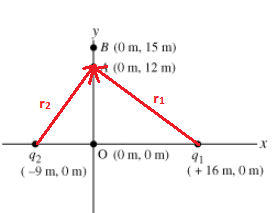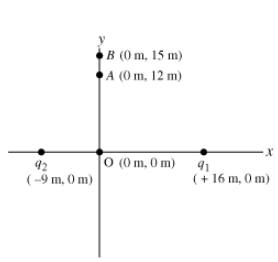# Problem: Two point charges are placed on the x-axis. The first charge, q1 = 8.00 nC, is placed a distance 16.0 m from the origin along the positive x-axis; the second charge, q2 = 6.00 nC, is placed a distance 9.00 m from the origin along the negative x-axis.(a) Calculate the electric field at point A, located at coordinates (0 m, 12.0 m).(b) Give the x and y components of the electric field as an ordered pair.Express your answer in newtons per coulomb to three significant figures.EAx, EAy =

###### FREE Expert Solution

Electric field,

$\overline{){\mathbf{E}}{\mathbf{=}}\frac{\mathbf{k}\mathbf{q}}{{\mathbf{r}}^{\mathbf{2}}}}$

Vector magnitude:

$\overline{)\mathbf{|}\stackrel{\mathbf{⇀}}{\mathbf{r}}\mathbf{|}{\mathbf{=}}\sqrt{{{\mathbf{r}}_{\mathbf{x}}}^{\mathbf{2}}\mathbf{+}{{\mathbf{r}}_{\mathbf{y}}}^{\mathbf{2}}}}$

Direction for angles measured from the x-axis:

Resolving vector components:

a)r1 = (-16.0 i + 12 j ) m (the vector moves in the negative x-direction)

$\begin{array}{rcl}\mathbf{|}{\stackrel{\mathbf{⇀}}{\mathbf{r}}}_{\mathbf{1}}\mathbf{|}& \mathbf{=}& \sqrt{{{\mathbf{r}}_{\mathbf{1}\mathbf{x}}}^{\mathbf{2}}\mathbf{+}{{\mathbf{r}}_{\mathbf{1}\mathbf{y}}}^{\mathbf{2}}}\\ & \mathbf{=}& \sqrt{{\mathbf{\left(}\mathbf{-}\mathbf{16}\mathbf{.}\mathbf{0}\mathbf{\right)}}^{\mathbf{2}}\mathbf{+}{\mathbf{\left(}\mathbf{12}\mathbf{.}\mathbf{0}\mathbf{\right)}}^{\mathbf{2}}}\end{array}$

|r1| = 20 m

θ1 = tan-1 (r1y/r1x) = tan-1 (12/-16) = -36.9° (negative x-component shows that the angle is above the the negative x-axis).

From the positive x-axis:

θ1 = 180 - 36.9 = 143.1

r2 = (9 i + 12 j) m (the vector moves in the positive x-direction)

98% (196 ratings)###### Problem Details

Two point charges are placed on the x-axis. The first charge, q1 = 8.00 nC, is placed a distance 16.0 m from the origin along the positive x-axis; the second charge, q2 = 6.00 nC, is placed a distance 9.00 m from the origin along the negative x-axis.

(a) Calculate the electric field at point A, located at coordinates (0 m, 12.0 m).

(b) Give the x and y components of the electric field as an ordered pair.

EAx, EAy =